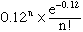I am the father of a high school student who has a science project. Although I am reasonable at math, I was never very good at statistics and have come up against a problem that I cannot solve. It is necessary to complete a science project. Assume: Leukemia occurs at an incidence of 1 in 10,000. Benzene is a known carcinogen which causes Leukemia and has been found in toxic levels in the homes of a community of 1200 people. Question: How many cases of Leukemia would be necessary to reject the null hypothesis. That is, to suggest that statistically, the cases are more likely due to benzene exposure rather than chance. Thank you, in advance, for your help on this problem. Don Hi Don, This is a "textbook question" -- it has little to do with with the way leukemia is distributed. The probability that we choose a community at random and it has one instance of leukemia is already "very small" -- If (contrary to fact) the the disease hit everybody with probability equal to 1/10000, then the probability of exactly n instances in a community of 1200 is(the Poisson probability with mean 1200/10000). The probability of no cases is about 0.89. The probability of exactly 1 case is only about 0.11. The probability of more than one case 1 - [ prob(n = 0) + prob(n = 1)] is about 0.007. Of more than 2 cases is 0.0003. On the other hand, there is something wrong here. When this sort of analysis is made, the community has not been randomly chosen. We have no idea what the probability would be for a nonrandomly chosen community: there are just too many factors involved beyond benzene. All one can say is that it is very unlikely that there would be three or more cases in so small a community, so that chance variation would not be the best way to explain a number of observed cases greater than 2. One would have to eliminate dozens of other explanations before concluding that benzene is the likely cause. You could use this kind of probabilistic analysis to argue that a serious scientific study should be undertaken but a statistical hypotheses test is not appropriate here. Chris and Penny Go to Math Central